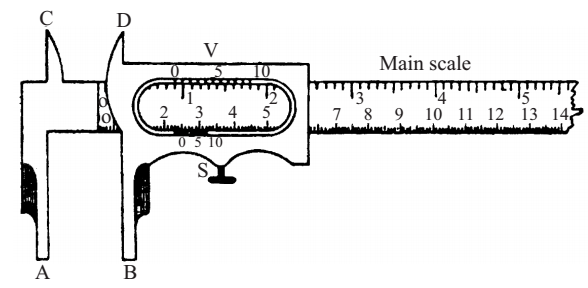# Vernier Callipers Experiment

Experiment: Determine the internal diameter and depth of a cylindrical container (like tin can, calorimeter) using a vernier callipers and find its capacity. Verify the result using a graduated cylinder.

Volume of Cylinder

The volume of a cylinder is given by the relation:

V = πr2h = π(d/2)2h = 1/4 × πd2h

where

• d = internal diameter of cylinder
• r = internal radius of the cylinder
• h = depth of cylinder

Material Required

A vernier callipers, a calorimeter, a graduated cylinder, a glass slab

### Vernier Callipers

Vernier callipers consists of a pair of calliperc having a vernier and main scale arrangement. The instrument has two jaws A and B. The vernier scale can easily slide along the edge of the main scale. The graduations of the vernier scale are so designed that a certain number of divisions of vernier scale, say 10, are coincident to 9 number of division of main scale.The difference between one smallest division of the main scale and one division of the vernier scale is known as vernier constant and is also the least count of the vernier device.

### A. To Find least count or vernier constant

1. Observe the divisions on the vernier scale are smaller than those on the main scale. The difference between one main scale division and one vernier division is called vernier constant or least count of the vernier callipers.

2. Observe the number of vernier divisions (n) which match against one less number of divisions of main scale (n-1).

3. Calculate the least count:

1 division of vernier scale = (n-1)/n division of main scale

Least count = 1 main scale division – 1 vernier scale division

= 1 main scale division – (n-1)/n main scale division

= 1/n main scale division

### B. To find the zero error of the vernier scale

4. With the jaws of the callipers closed, if the zero marks of the main scale does not coincide with the zero mark of the vernier scale, the instrument has a zero error. If the zero mark of the vernier scale is on the left of the main scale’s zero mark then the zero error is negative and when it is on the right of the main scale’s zero mark the zero error is positive.

5. If there is zero error, observe which vernier scale (v.s.) division best coincides with any main scale (ms) division with jaws of the callipers closed. The value of the zero error is the product of the best coinciding vernier division and least count of the vernier callipers when zero error is positive. On the other hand when zero error is negative, the coinciding vernier division is to be seen from the end of the vernier scale, backwards.

6. While observing which vernier scale division coincides with a main scale division, it may happen that none coincides. For example, 5th may be a little ahead and 6th may be a little before a main scale division. Observe, which one is closest to a main scale division.

### C. To find the zero correction of the vernier scale

7. It is the negative of the zero error.

Zero correction = – (zero error)

Zero correction is added algebraically in the observed diameter to get the corrected diameter.

### D. Measuring internal diameter

8. To measure the internal diameter of the calorimeter, place the vernier callipers with the upper jaws inside the calorimeter. The upper jaws of the vernier callipers should firmly touch the ends of a diameter of the calorimeter, but without deforming the calorimeter.

9. Note the main scale reading immediately before the zero mark of the vernier and also note the division of the vernier which coincides with any of the main scale divisions.

10. Since the calorimeter may not be of precisely circular shape, take one more observation along a diameter perpendicular to previous one.

11. Repeat the pair of observations at least three times and record them.

### E. Measuring depth

Next, let the end of the vernier callipers stand on its end on a glass slab, push down its depth gauge (the central moving strip), so that it also firmly touches the glass slab. Then note the zero error of its depth gauge.

12. Next, set the vernier callipers with its end resting on the upper edge of the calorimeter and its depth gauge touching the bottom inside. Thus note the observed depth of the calorimeter. Calculated corrected depth by applying zero correction.

### F. Verification

13. Next, in order to verify the capacity of calorimeter measured by vernier callipers, fill it completely with water. Pour this water in to an empty graduated cylinder and observe the volume of this water. Both values should be in agreement within experimental error.

### Sources of Errors

1. None of the vernier divisions may be exactly coincident with a main scale division.
2. The vernier scale may be loose,.and the caliberation may not be uniform. Similarly, vernier jaws may not be at right angles to its main scale. These are common small defects in cheaper instruments.